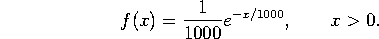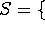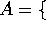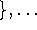M362K First Midterm Exam, February 7, 2002

Problem 1. Light Bulbs

Suppose the probability function describing the life (in hours) of an ordinary 60 Watt bulb isLet A be the event that the bulb lasts 500 hours or more, and let B be the event that it lasts between 400 and 800 hours.

a) What is the probability of A?

b) What is the probability of B?

c) What is P(A|B)?

Problem 2. Lawyers and Liars (20 points)

A fashionable club has 100 members, 30 of whom are lawyers. 25 of the members are liars, while 55 are neither lawyers nor liars.

a) How many of the lawyers are liars?

b) A liar is chosen at random. What is the probability that he is a lawyer?

Problem 3. Spring Break Sports

I haven't decided what to do over spring break. There is a 50% chance that I'll go skiing, a 30% chance that I'll go hiking, and a 20% chance that I'll stay home and play soccer. The (conditional) probability of my getting injured is 30% if I go skiing, 10% if I go hiking, and 20% if I play soccer.

a) What is the probability that I will get injured over spring break?

b) If I come back from vacation with an injury, what is the probability that I got it skiing?

Problem 4. Flipping Coins (30 points)

Suppose you flip a nickel, a dime and a quarter. Each coin is fair, and the flips of the different coins are independent. Let A be the event ``the total value of the coins that came up heads is at least 15 cents''. Let B be the event ``the quarter came up heads''. Let C be the event ``the total value of the coins that came up heads is divisible by 10 cents''.

a) Write down the sample space, and list the events A, B, and C. Your answer should be of the form ``(list of elements)(shorter list of elements)''.

b) Find P(A), P(B) and P(C).

c) Compute P(B|A).

d) Are B and C independent? Explain.